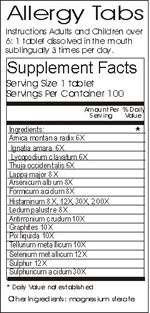Logic puzzles, riddles, math puzzles and brainteasers - pzzls.com

Vandaag is het 26 September 2023

Sort - Oldest on top
Page: << 1 2 3 4 5 >>

# Thunder and lightning - science puzzle

Difficulty:Rating: 3.2/5.0

 Why does a flash of lightning take only an instant, while the corresponding thunder can last for a few seconds? You might have expected that that the sound is caused by the flash, and that the thunder should therefore be audible also for an instant.# Quack medicine? - science puzzle

Difficulty:Rating: 2.8/5.0

 According to homeopaths the effect of a homeopathic medicin is indicated by a dilution factor. Their theory is that if a substance is diluted more, the effect of it will increase. The dilution factor of a homeopathic medicin consists of a number and a character. An X or D indicates that the substance is diluted a number times 10. A C stands for a factor 100. As an example, 6 X indicates that the substance is diluted 10^6 = 1,000,000 times. Typical dilution factors of homeopathic medicins lie between 6 X and 30 X. Suppose you bought a litre of homepathic medicin with a dilution factor of 30 X. Assume that water was used for the dilution proces, and that the molecules of the substance are about as big as the water molecules (smaller is almost impossible, since water is one of the smallest molecules). How many molecules of the substance can you find in your bottle. And how many litres would you need to buy to be sure that you will have at least one molecule of the substance?# Voltage voodoo - science puzzle

Difficulty:Rating: 3.1/5.0

 In the figure, an electrical circuit is drawn. In the middle of the circuit a infinitely long metal bar is placed indicated with the grey circle. In this bar, an oscillating magnetic field is induced by a coil which is attached somewhere to the bar. Two resistors are located in the circuit with values R1 and R2. The alternating voltages V1 and V2 are being measured with two perfect alternating voltages meters. What is the relation between the two voltages V1 and V2? a) V1 : V2 = R2 : R1 b) V1 : V2 = 1 : 1 c) V1 : V2 = R1 : R2 d) V1 = V2 = 0 e) something different.